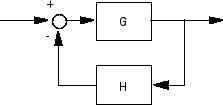inv

Invert models

inv
inv(sys,'min')

Description

inv inverts the input/output relation

$y=G\left(s\right)u$

to produce the model with the transfer matrix $H\left(s\right)=G{\left(s\right)}^{-1}$.

$u=H\left(s\right)y$

This operation is defined only for square systems (same number of inputs and outputs) with an invertible feedthrough matrix D. inv handles both continuous- and discrete-time systems.

inv(sys,'min') inverts sys to eliminate the extra states and obtain a model with as many states as sys or A respectively. For ss, genss and uss models, the inverse model is returned in implicit form by default. This option is ignored for sparse models because it typically destroys sparsity. Use isproper or ss(sys,'explicit') to extract an explicit model if desired.

Examples

Consider

$H\left(s\right)=\left[\begin{array}{cc}1& \frac{1}{s+1}\\ 0& 1\end{array}\right]$

At the MATLAB® prompt, type

H = [1 tf(1,[1 1]);0 1]
Hi = inv(H)

to invert it. These commands produce the following result.

Transfer function from input 1 to output...
#1:  1

#2:  0

Transfer function from input 2 to output...
-1
#1:  -----
s + 1

#2:  1

You can verify that

H * Hi

is the identity transfer function (static gain I).

Limitations

Do not use inv to model feedback connections such asWhile it seems reasonable to evaluate the corresponding closed-loop transfer function ${\left(I+GH\right)}^{-1}G$ as

inv(1+g*h) * g

this typically leads to nonminimal closed-loop models. For example,

g = zpk([],1,1)
h = tf([2 1],[1 0])
cloop = inv(1+g*h) * g

yields a third-order closed-loop model with an unstable pole-zero cancellation at s = 1.

cloop

Zero/pole/gain:
s (s-1)
-------------------
(s-1) (s^2 + s + 1)

Use feedback to avoid such pitfalls.

cloop = feedback(g,h)

Zero/pole/gain:
s
-------------
(s^2 + s + 1)
Introduced before R2006a